# nLab tensor network

Contents

### Context

#### Monoidal categories

monoidal categories

# Contents

## Idea

The term tensor network has become popular in quantum physics for essentially what in mathematical physics is known as Penrose notation and in monoidal category theory is referred to as string diagrams (see BCJ 10 for a bilingual account).

The term rose to prominence in quantum physics partly with discussion of finite quantum mechanics in terms of dagger-compact categories but mainly via its use for representing highly entangled states in the discussion of renormalization of non-perturbative effects in solid state physics (Swingle 09, Swingle 13) and the resulting discovery of the relation to holographic entanglement entropy and thus to the AdS/CFT correspondence.

In this context, a tensor network is a string diagram with an attitude, in that it is (just) a string diagram (typically regarded in the monoidal category of finite-dimensional vector spaces, but possibly also in super vector spaces etc.), but with:

1. the tensor product of all its external objects regarded as a space of states of a quantum system;

2. the element in that tensor product defined by the string diagram regarded as a state (wave function) of that quantum system.

For instance, if $\mathfrak{g}$ is a metric Lie algebra (with string diagram-notation as shown there), and with each tensor product-power of its dual vector space regarded as a Hilbert space, hence as a space of quantum states, via the given inner product on $\mathfrak{g}$, then an example of a tree tensor network state is the following: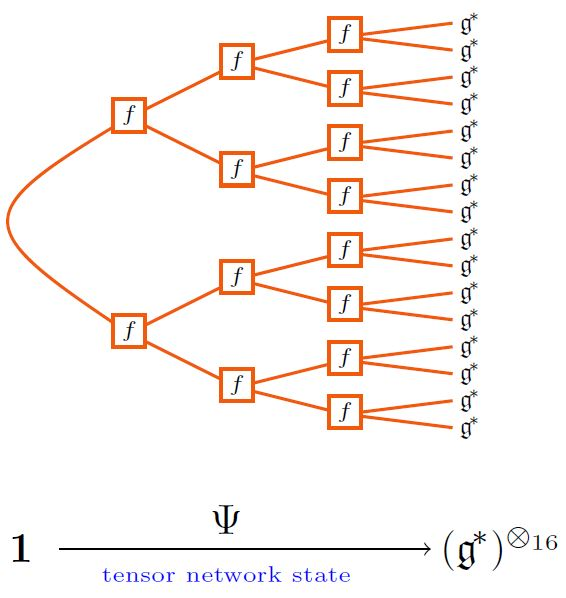graphics from Sati-Schreiber 19c

The quantum states arising this way are generically highly entangled: roughly they are the more entangled the more vertices there are in the corresponding tensor network.

The following is an example of what is called a matrix product state: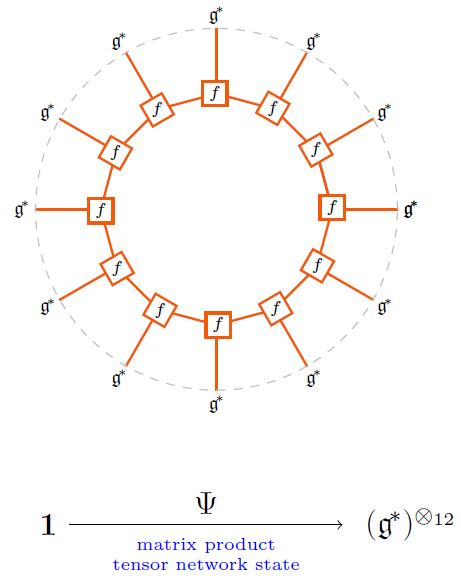graphics from Sati-Schreiber 19c

The tree tensor network states in the form of Bruhat-Tits trees play a special role in the AdS/CFT correspondence, either as

1. a kind of lattice QFT-approximation to an actual boundary CFT quantum state,

3. as a reflection of actual crystal-site quantum states in AdS/CFT in solid state physics: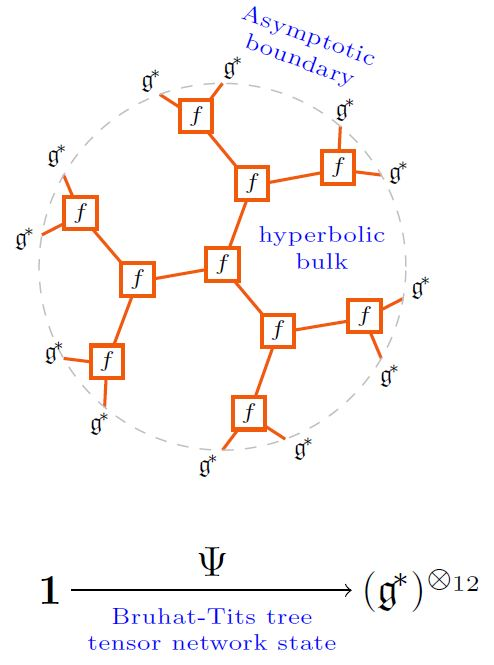graphics from Sati-Schreiber 19c

(As in HMSS 16, HLM 19. But maybe one wants the Poincaré-dual networks, instead, as in HMPS 18?)

For tensor network states dual to tesselations of hyperbolic space and made from perfect tensors one finds that the entanglement entropy of the tensor subspace associated with an interval on the boundary becomes proportional, for large number of vertices, to the hyperbolic bulk boundary length of the segment of the tree network that ends on this interval, according to the Ryu-Takayanagi formula (PYHP 15, Theorem 2). Hence one speaks of holographic entanglement entropy. For more on this see below.

General tensor network states are mixtures of the above examples. For instance in … is the suggestion that BTZ black holes are encoded by networks that look like Bruhat-Tits trees towards the boundaries and like matrix product states towards the interior: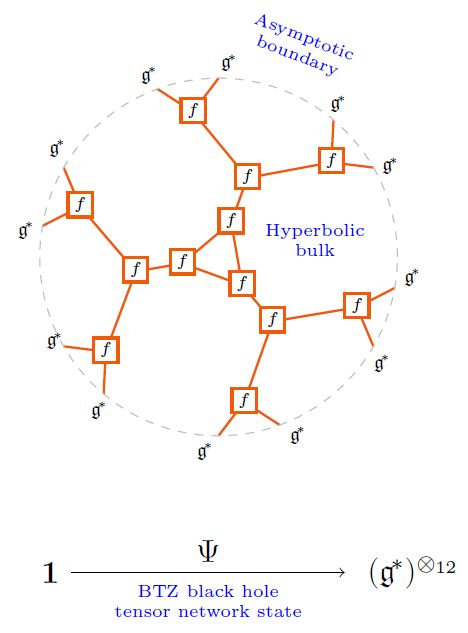(As in ESZ 19.)

## For holographic entanglement entropy

under construction

Appearance in renormalization: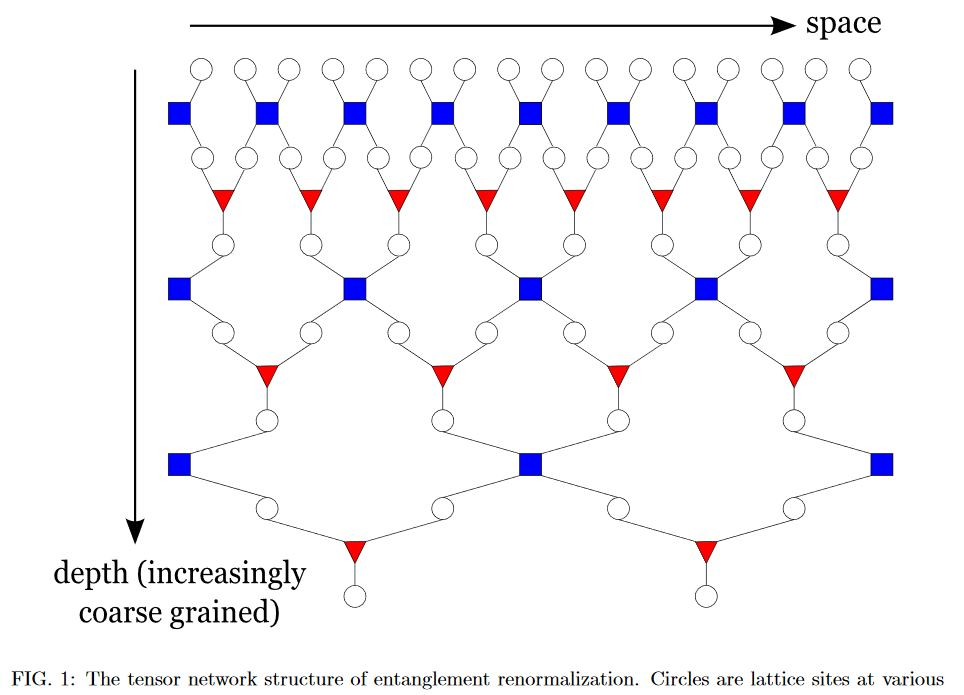from Swingle 09

Application to holographic entanglement entropy (…)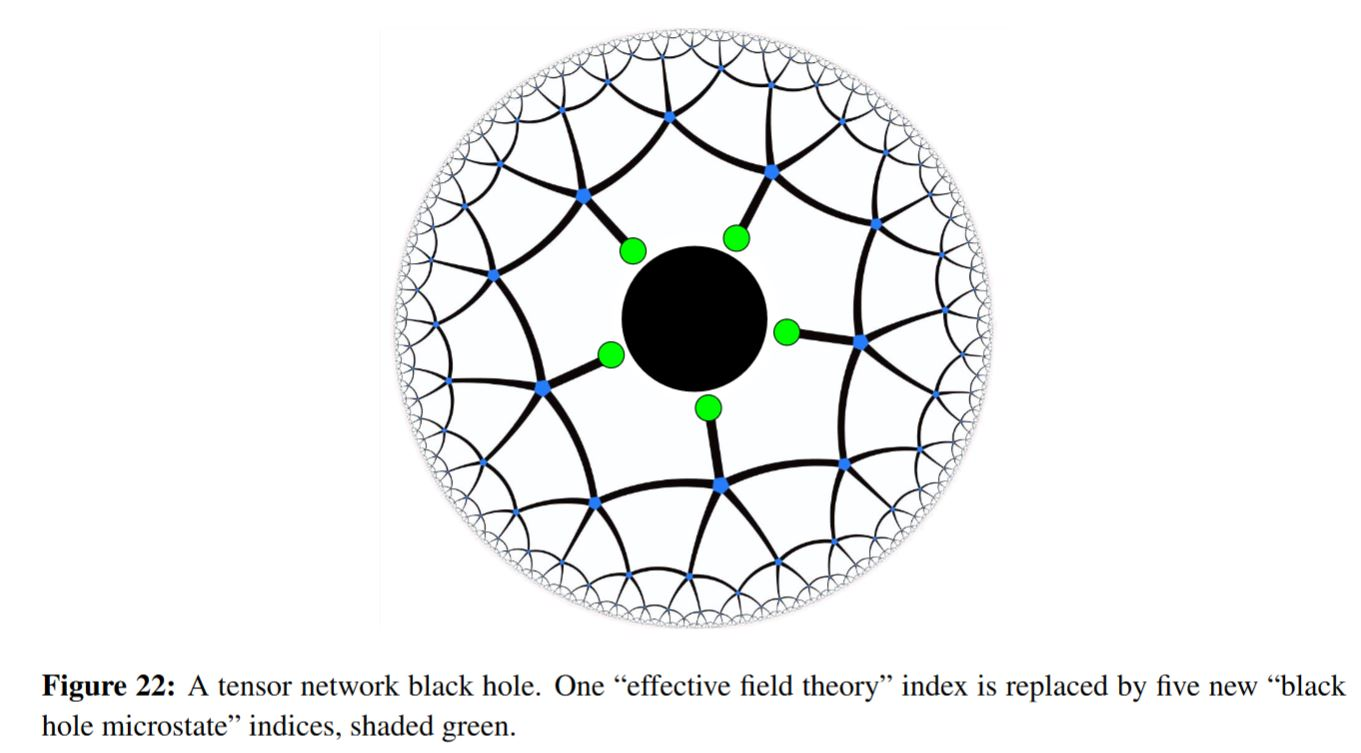graphics grabbed from Harlow 18, see at HaPPY code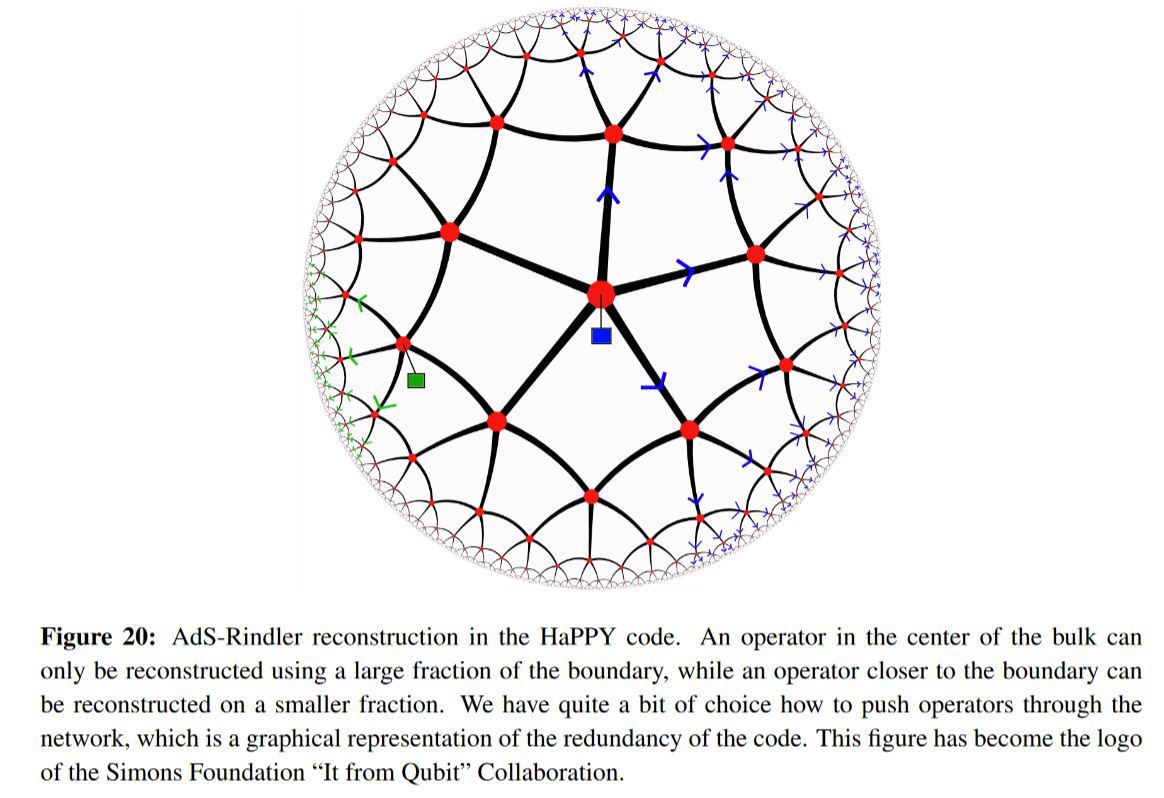graphics grabbed from Harlow 18, see at HaPPY code

In this context the Ryu-Takayanagi formula for holographic entanglement entropy has an exact proof PYHP 15, Theorem 2.

## Examples

### General

Review and exposition:

With global symmetry:

• Sukhwinder Singh, Robert N. C. Pfeifer, Guifre Vidal, Tensor network decompositions in the presence of a global symmetry, Phys. Rev. A82:050301, 2010 (arXiv:0907.2994)

• Sukhwinder Singh, Robert N. C. Pfeifer, Guifre Vidal, Tensor network states and algorithms in the presence of a global $U(1)$ symmetry, Phys. Rev. B 83, 115125 (2011) (arXix:1008.4774)

• Sukhwinder Singh, Guifre Vidal, Tensor network states and algorithms in the presence of a global $SU(2)$ symmetry, Phys. Rev. B 86, 195114 (2012) (arXiv:1208.3919)

Further resources:

### Application in solid state physics

Discussion of solid state physics via tensor network states:

General:

Concrete materials:

• A. Kshetrimayum, C. Balz, B. Lake, Jens Eisert, Tensor network investigation of the double layer Kagome compound $Ca_{10} Cr_{7} O_{28}$ (arXiv:1904.00028)

### Relation to (topological) phases of matter

Classification of (topological) phases of matter via tensor network states:

• C. Wille, O. Buerschaper, Jens Eisert, Fermionic topological quantum states as tensor networks, Phys. Rev. B 95, 245127 (2017) (arXiv:1609.02574)

• Andreas Bauer, Jens Eisert, Carolin Wille, Towards a mathematical formalism for classifying phases of matter (arXiv:1903.05413)

### In holographic entanglement entropy

The use of tensor networks as a tool in holographic entanglement entropy goes back to

Review in

• Frank Verstraete, J. I. Cirac, V. Murg, Matrix Product States, Projected Entangled Pair States, and variational renormalization group methods for quantum spin systems, Adv. Phys. 57,143 (2008) (arXiv:0907.2796)

### In the $p$-adic AdS/CFT correspondence

• Matthew Heydeman, Matilde Marcolli, Ingmar Saberi, Bogdan Stoica, Tensor networks, $p$-adic fields, and algebraic curves: arithmetic and the $AdS_3/CFT_2$ correspondence (arXiv:1605.07639)

• Arpan Bhattacharyya, Ling-Yan Hung, Yang Lei, Wei Li, Tensor network and ($p$-adic) AdS/CFT, JHEP 1801 (2018) 139 (arXiv:1703.05445)

• Ling-Yan Hung, Wei Li, Charles M. Melby-Thompson, $p$-adic CFT is a holographic tensor network (arXiv:1902.01411)

• Lin Chen, Xirong Liu, Ling-Yan Hung, Emergent Einstein Equation in p-adic CFT Tensor Networks (arXiv:2102.12022)

• Lin Chen, Xirong Liu, Ling-Yan Hung, Bending the Bruhat-Tits Tree I:Tensor Network and Emergent Einstein Equations (arXiv:2102.12023)

• Lin Chen, Xirong Liu, Ling-Yan Hung, Bending the Bruhat-Tits Tree II: the p-adic BTZ Black hole and Local Diffeomorephism on the Bruhat-Tits Tree (arXiv:2102.12024)

Discussion of BTZ black holes via tensor networks in the p-adic AdS/CFT correspondence:

### As quantum error correcting codes

Further interpretation of tensor networks in terms of quantum error correcting codes:

• Andrew J. Ferris, David Poulin, Tensor Networks and Quantum Error Correction, Phys. Rev. Lett. 113, 030501 (2014) (arXiv:1312.4578)

and specifically in the HaPPY code modelling holographic entanglement entropy:

reviewed in

Generalization to the Majorana dimer code:

reviewed in:

Discussion of asymptotic boundaries of hyperbolic tensor networks as quasicrystals:

• Latham Boyle, Madeline Dickens, Felix Flicker, Conformal Quasicrystals and Holography, Phys. Rev. X 10, 011009 (2020) (arXiv:1805.02665)

### Relation to spin chains

Relation to spin chains:

• Mari Carmen Banuls, Michal P. Heller, Karl Jansen, Johannes Knaute, Viktor Svensson, From spin chains to real-time thermal field theory using tensor networks (arXiv:1912.08836)

### Application to lattice gauge theory

Application to lattice gauge theory:

• Luca Tagliacozzo, Alessio Celi, Maciej Lewenstein, Tensor Networks for Lattice Gauge Theories with continuous groups, Phys. Rev. X 4, 041024 (2014) (arXiv:1405.4811)

• M.C. Bañuls, R. Blatt, J. Catani, A. Celi, J.I. Cirac, M. Dalmonte, L. Fallani, K. Jansen, M. Lewenstein, S. Montangero, C.A. Muschik, B. Reznik, E. Rico, Luca Tagliacozzo, K. Van Acoleyen, Frank Verstraete, U.-J. Wiese, M. Wingate, J. Zakrzewski, P. Zoller:

Simulating Lattice Gauge Theories within Quantum Technologies

### In higher parallel transport

Discussion in relation to higher parallel transport: